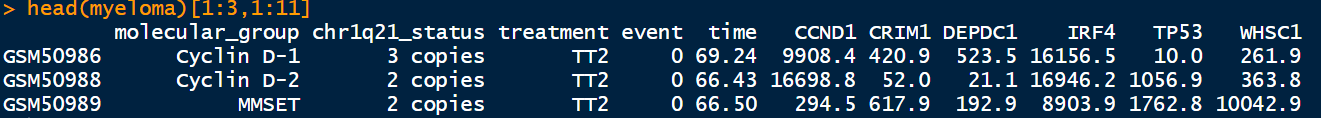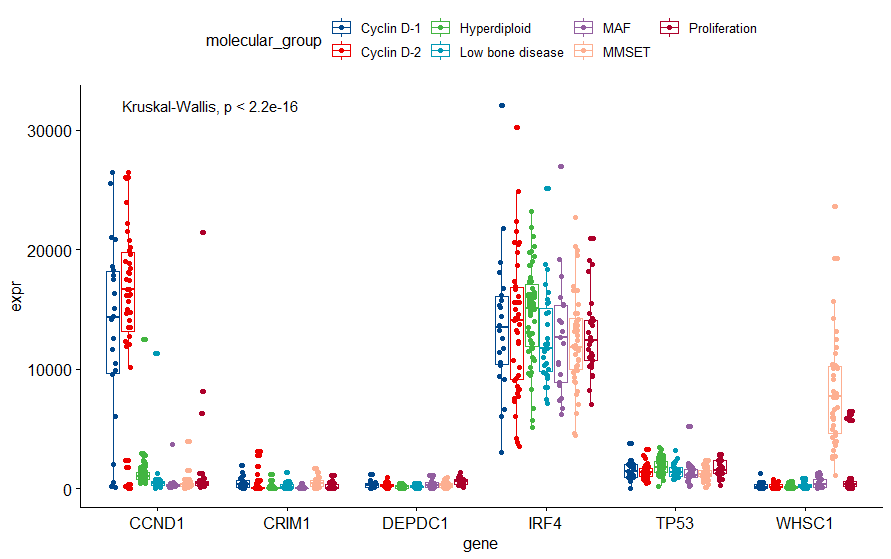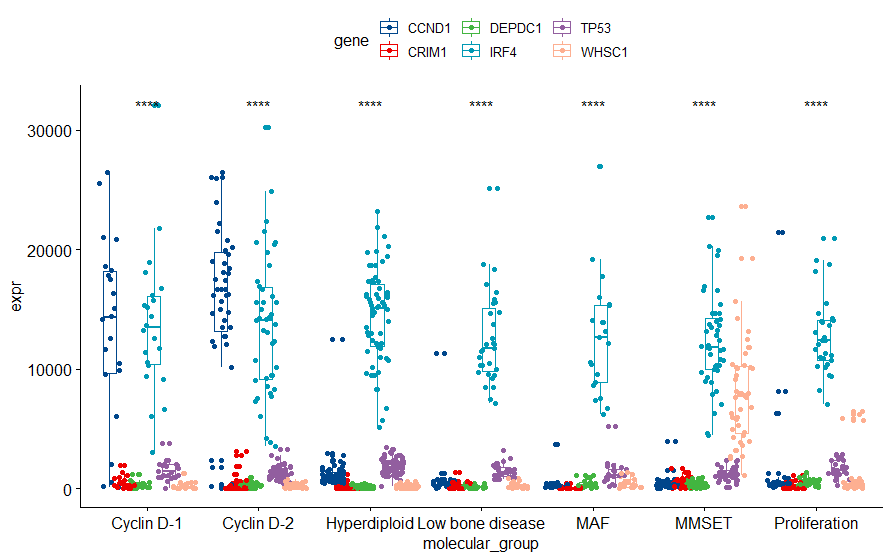Posted on

1. 为什么要进行数据长宽变换

2. 宽数据变长数据

``````library(survminer)

data("myeloma")``````> dim(myeloma)

 256 11``````

``````library(tidyr)

library(dplyr)

library(tibble)

myeloma_longer<- myeloma %>%

rownames_to_column(var = 'sample') %>%

pivot_longer( cols = c("CCND1":"WHSC1"),

names_to = 'gene',

values_to = 'expr')``````

``````> dim(myeloma_longer)

 1536 8

# A tibble: 6 x 8

sample molecular_group chr1q21_status treatment event time gene expr

<chr> <fct> <fct> <fct> <int> <dbl> <chr> <dbl>

1 GSM50986 Cyclin D-1 3 copies TT2 0 69.2 CCND1 9908.

2 GSM50986 Cyclin D-1 3 copies TT2 0 69.2 CRIM1 421.

3 GSM50986 Cyclin D-1 3 copies TT2 0 69.2 DEPDC1 524.

4 GSM50986 Cyclin D-1 3 copies TT2 0 69.2 IRF4 16156.

5 GSM50986 Cyclin D-1 3 copies TT2 0 69.2 TP53 10

6 GSM50986 Cyclin D-1 3 copies TT2 0 69.2 WHSC1 262.``````

``````p <- ggboxplot(myeloma_longer, x="gene", y="expr", color = "molecular_group", palette = "lancet", add = "jitter")

m1=p+stat_compare_means(aes(group=gene)) #默认是Wilcoxon

m1````````````p <- ggboxplot(myeloma_longer, x="molecular_group", y="expr", color = "gene", palette = "lancet", add = "jitter")

m1=p+stat_compare_means(aes(group=gene),label = "p.signif") #默认是Wilcoxon

m1``````10.12小更新：有些时候横坐标的项目比较多，直接横行排列，显得臃肿，加上一句代码，让横坐标转换角度，这样就使得横坐标清新了然。

``````p <- ggboxplot(myeloma_longer, x="molecular_group", y="expr", color = "gene", palette = "lancet", add = "jitter")+

rotate_x_text(angle = 40)``````

3. 长数据变宽数据

3.1无差错转换

3.2 出现错误提示

``````data <- iris[, c(4,5)]

data %>% pivot_wider(names_from = Species,

values_from = Petal.Width)``````

``````Warning message:

Values in `Petal.Width` are not uniquely identified; output will contain list-cols.

* Use `values_fn = list(Petal.Width = list)` to suppress this warning.

* Use `values_fn = list(Petal.Width = length)` to identify where the duplicates arise

* Use `values_fn = list(Petal.Width = summary_fun)` to summarise duplicates``````

``````data <- iris[c(4,5)]

dat <- data %>%

group_by(Species) %>%

mutate(index = row_number()) %>%

pivot_wider(names_from = Species,

values_from = Petal.Width) %>%

select(-index)

dat``````

``````> dat

# A tibble: 50 x 3

setosa versicolor virginica

<dbl> <dbl> <dbl>

1 0.2 1.4 2.5

2 0.2 1.5 1.9

3 0.2 1.5 2.1

4 0.2 1.3 1.8

5 0.2 1.5 2.2

6 0.4 1.3 2.1

7 0.3 1.6 1.7

8 0.2 1 1.8

9 0.2 1.3 1.8

10 0.1 1.4 2.5

# ... with 40 more rows``````